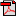# Lesson 2: Atomic Structure

## Activities

### Activity 1: Making a Basic Atomic Model

Materials: blank paper, compass (kit), construction paper (kit), glue stick, hole punch, ruler
In this activity, you will create a model of a fluorine atom and a sodium atom.

Note: For this activity, one important thing to recognize is that atoms that make up elements are not different for different states of matter. An atom for a solid is the same as an atom for a liquid or a gas of the same element. You will learn more about different states of matter in Lesson 4.

As you create your models, keep in mind that the atom has three parts — the neutron, the proton, and the electron — and that each part has a specific location:
• The protons and the neutrons are bundled together in the nucleus
• The electrons are in "orbit" around the nucleus
Each atom has layers around the nucleus called shells or orbitals. Each orbital can hold a certain number of electrons. For example, the shell closest to the nucleus is the 1st orbital (n = 1), and the maximum number of electrons it can contain is 2.

In the 2nd orbital (n=2), the maximum number of electrons is 8, and in the 3rd orbital (n=3), the maximum number of electrons is 18.

For example, the element oxygen (atomic symbol = O) has the following numbers:
• Electrons: 8
• Neutrons: 8
• Protons: 8
The nucleus of an oxygen atom contains 8 protons and 8 neutrons. Around the nucleus are 8 electrons — the first orbital contains two electrons, and the second orbital contains six. The total number of electrons = Two (2) + Six (6).

The "Sample Atomic Model" diagram for oxygen shows the nucleus with 8 protons and 8 neutrons, a shell around the nucleus with 2 electrons, and another shell with 6 electrons.
Sample Atomic ModelAs you create your own models, refer to the "Making a Basic Atomic Model" sheet for details.

Note: The sample atomic models you will create are not to scale. The goal of this activity is for you to develop the basic concept of the structure of an atom.Student Activity Page

#### Creating a Fluorine Model

Now you will create a model of a fluorine (atomic symbol = F) atom. Fluorine is a gas that has the following numbers of each particle (use the colors in parentheses for your model):
• Electrons: 9 (black)
• Neutrons: 10 (gray)
• Protons: 9 (another color)
Follow these directions to make your own model of a fluorine atom:
1. Use a hole punch and the specified colors of construction paper to punch out the correct number of electrons, neutrons, and protons for fluorine.
2. On a sheet of blank paper, use a compass to draw a circle with a 4 inch diameter (2 inch radius). This circle represents the nucleus of the atom. (Be sure that the protons and neutrons all fit within the nucleus. Although protons and neutrons can touch, you may need to make your circle bigger if they are too crowded.)
3. Use a glue stick to attach the protons and neutrons within the nucleus.
4. Using the compass, draw a second circle larger than the first. (You should have about one-half inch between the two lines.)
5. Use the glue stick to affix the proper number of electrons on this circle; this is the 1st orbital (n=1).
6. Using the compass, draw a third circle larger than the second, leaving about one-half inch between this line and the 1st orbital.
7. Put the proper number of electrons on this circle; this is the 2nd orbital (n=2).

#### Creating a Sodium Model

Next, create a model of an atom of sodium (chemical symbol = Na). Sodium is a solid. It has the following number of each particle:
• Electrons: 11
• Neutrons: 12
• Protons: 11Student Activity Page